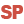HelpWLanguageWLanguage functionsStandard functionsEncryption/compression functionsEncryptStandardPresentationExampleResults obtained with AES encryptionRelated examplesSee also.CrypteStandardAsymmetricCryptDecryptAsymmetricDecryptAsymmetricEnclosedEncryptedAsymmetricCompressCompression / encryption functionsConstants for encryption/compressionDecodeDecrypt (Example)DecryptDecryptAsymmetricDecryptAsymmetricEnvelopedDecryptStandard (Example)DecryptStandardEncodeEncrypt (Example)EncryptEncryptAsymmetricEncryptAsymmetricEnvelopedEncryptGenerateRSAKeyEncryptStandard (Example)EncryptStandardLuhnCalculateLuhnCheckUUDecodeUUEncodeUncompress
• Results obtained with AES encryption
WINDEVWEBDEVWINDEV MobileOthersWarning
From version 24, CryptStandard is kept for backward compatibility. This function is replaced by EncryptStandard.
Encrypts a character string or a binary buffer by using a symmetrical encryption algorithm (AES, DES, etc.). This encrypted message can be decrypted by DecryptStandard.
Unlike Encrypt and Decrypt, EncryptStandard and DecryptStandard use the standard encryption algorithms that allow you to exchange encrypted messages between different runtime platforms (Windows, Linux, Android, Java, iOS, PHP, etc.) and/or with external tools.
Remark: The first call to EncryptStandard can be quite long because the randomization must be initialized.
// Encrypt a character string by using the AES algorithm
// ------------------------------------------------------------------
bufMessage is Buffer = "Message to encrypt"
bufKey is Buffer = HashString(HA_MD5_128, "password")
bufEncrypt is Buffer = EncryptStandard(bufMessage, bufKey, cryptAES128)

// Decrypt in WLanguage
-------------------------
bufResult is Buffer = DecryptStandard(bufEncrypt, bufKey, cryptAES128)
Info(bufResult)
// Encryption in WLanguage
// ---------------------
bufMessage is Buffer = "Message to encrypt"
bufKey is Buffer = HashString(HA_MD5_128, "password")
bufEncrypt is Buffer = EncryptStandard(sMessage, bufKey, cryptAES128)

//---------------------------------------------------------------
// Decrypt via a call to the Mcrypt API of PHP
nSizeIV is int = 128 / 8
bufIV is Buffer = bufEncrypt[[ TO nSizeIV]]
bufData is Buffer = bufEncrypt[[nSizeIV+1 TO]]

EXTERN mcrypt_decrypt//PHP API (to be done in a WEBDEV PHP server process)
Trace(mcrypt_decrypt( "rijndael-128", bufKey, bufData, "cbc", bufIV))
Syntax
<Result> = EncryptStandard(<Message> , <Key> [, <Algorithm> [, <Operation mode> [, <Padding> [, <Initialization vector>]]]])
<Result>: Binary buffer
• Result of encryption for the specified message,
• Empty string ("") if an error occurred. To get more details on the error, use ErrorInfo.
This buffer includes two sections:
• The initialization vector (IV) which corresponds to a randomly generated block of bits (or specified with <Initialization vector>) that has been combined with the first block of encrypted data. This vector is required to allow the message decryption. Its size corresponds to the size of blocks used by the encryption algorithm (see the <Algorithm> parameter).
• The encrypted data.
If the message must be decrypted:
• by DecryptStandard, all you have to do is pass the entire buffer to the function.
• by an external tool, the buffer must be split to separate the initialization vector from the encrypted data (see the example).
Remark: If the specified mode is cryptECB, no initialization vector is used, and in this case the function returns the encrypted data directly.
<Message>: Binary buffer
Message to encrypt.
<Key>: Buffer
Key with which the data must be encrypted. The size of this key depends on the encryption algorithm used. The key size must correspond to the one of the algorithm.
We recommend that you use the hashing functions (HashString for example) to create a key from a password. A long password that includes several alphanumeric characters and distinct symbols provides better encryption security.
Cross-platform development: To handle character string, the same format must be used on all the platforms. We advise you to use strings in UTF 8 format (and to convert the Unicode strings if necessary).
<Algorithm>: Optional Integer constant
Encryption algorithm to use:
 crypt3DES Triple Data Encryption Standard. Size of key: 192 bits. Size of blocks: 64-bit.Size of initialization vector (IV): 64-bit. cryptAES128(Default value) Advanced Encryption Standard. Size of key: 128 bits. Size of blocks: 128 bits. Size of initialization vector (IV): 128 bits. cryptAES256 Advanced Encryption Standard. Size of key: 256 bits. Size of blocks: 128 bits. Size of initialization vector (IV): 128 bits. cryptDES Data Encryption Standard. Size of key: 64-bit. Size of blocks: 64-bit. Size of initialization vector (IV): 64-bit.Caution: this algorithm is currently deprecated.
<Operation mode>: Optional Integer constant
Process mode of blocks by the encryption algorithm:
 cryptCBC (Default value) Cipher Block Chaining - Sequence of blocks cryptCFB Cipher Feedback - Feedback encryption. This process mode is available if the algorithm used corresponds to the cryptAES256 constant. cryptCTR Cipher Counter - Encryption based on a counter. This process mode is available if the algorithm used corresponds to the cryptAES256 constant. cryptECB Electronic Code Book - Dictionary of codes. This operation mode is not recommended and it should only be used for compatibility reasons.No initialization vector is used, and in this case the function returns the encrypted data directly.
Padding mode for encrypted data to be compatible with the size required by block encryption algorithms:
 cryptPaddingPKCS (Default value) The data is filled with bytes whose value corresponds to the total number of bytes added to reach the requested size. cryptPaddingZero The data is filled with binary zeros until the requested size is reached.
<Initialization vector>: Optional buffer
Initialization vector (IV) to be used for encryption. The size of the buffer depends on the encryption algorithm (<Algorithm< parameter). If this parameter is not specified, the initialization vector is randomly generated.
Caution: This parameter should only be used if you need a custom initialization vector in your project. Otherwise, it is strongly advised to keep the generation of the initialization vector in random mode.
Remarks

Results obtained with AES encryption

Encryption performed with an AES algorithm uses an initialization vector. This initialization vector is modified each time the function is called. It is therefore normal to get a different result each time the same information is encrypted. However, the expected value is correctly retrieved when DecryptStandard is called.
Related Examples:Unit examples (WINDEV): The encryption functions [ + ] Using the encryption/decryption functions of WINDEV.This example is used to:- Encrypt a character string- Decrypt a character string
Component: wd280com.dll
Minimum version required
• Version 20